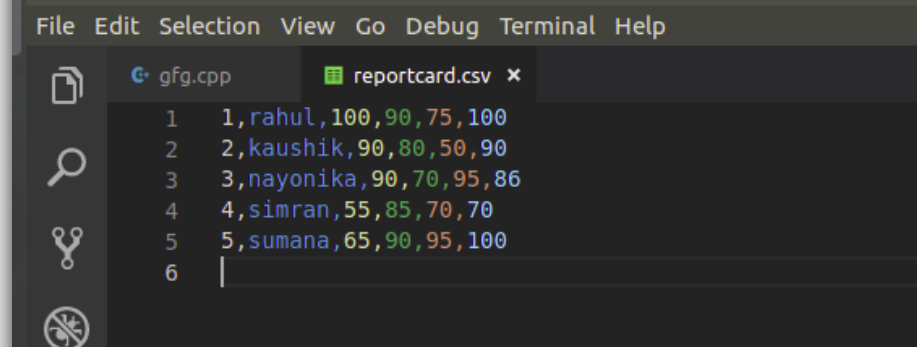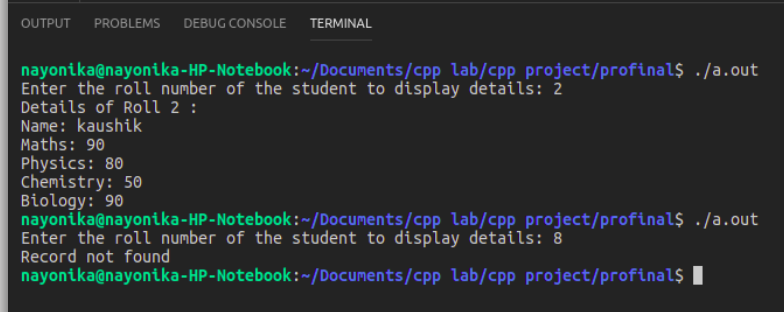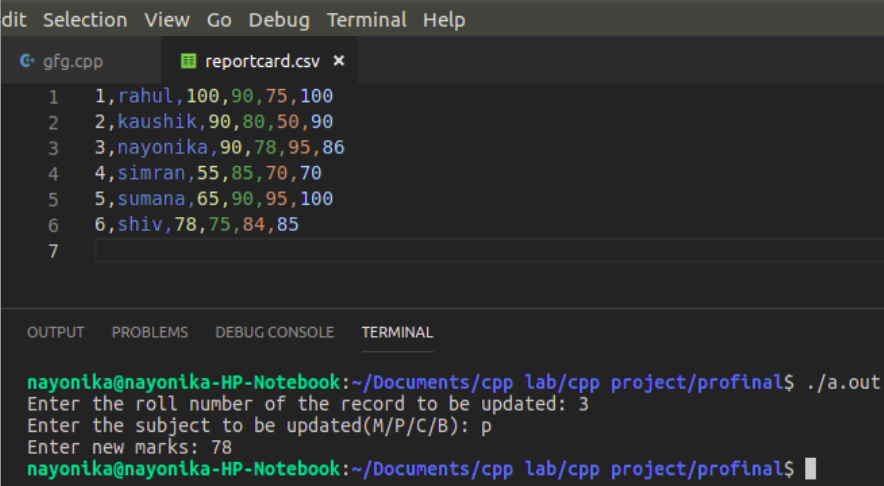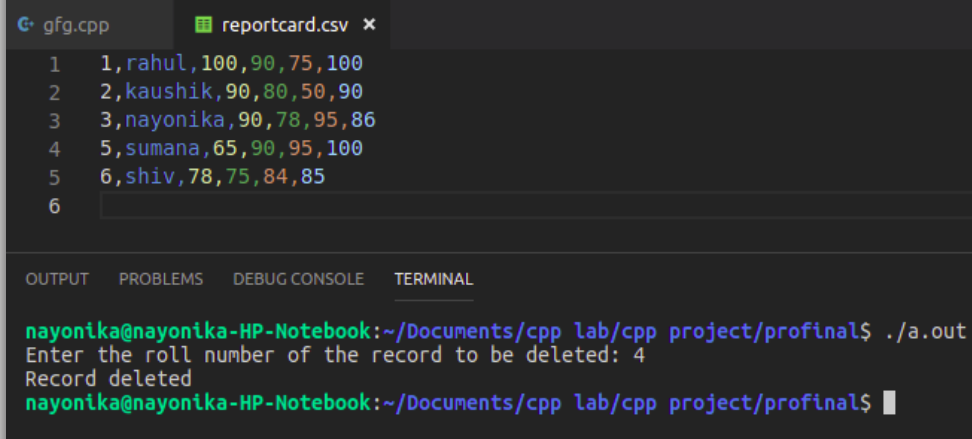Related Articles
CSV file management using C++
• Difficulty Level : Medium
• Last Updated : 25 Jun, 2020

CSV is a simple file format used to store tabular data such as a spreadsheet or a database. CSV stands for Comma Separated Values. The data fields in a CSV file are separated/delimited by a comma (‘, ‘) and the individual rows are separated by a newline (‘\n’). CSV File management in C++ is similar to text-type file management, except for a few modifications.

This article discusses about how to create, update and delete records in a CSV file:

Note: Here, a reportcard.csv file has been created to store the student’s roll number, name and marks in math, physics, chemistry and biology.

1. Create operation:

The create operation is similar to creating a text file, i.e. input data from the user and write it to the csv file using the file pointer and appropriate delimiters(‘, ‘) between different columns and ‘\n’ after the end of each row.

## CREATE

 `void` `create()``{``    ``// file pointer``    ``fstream fout;`` ` `    ``// opens an existing csv file or creates a new file.``    ``fout.open(``"reportcard.csv"``, ios::out | ios::app);`` ` `    ``cout << ``"Enter the details of 5 students:"``         ``<< ``" roll name maths phy chem bio"``;``    ``<< endl;`` ` `    ``int` `i, roll, phy, chem, math, bio;``    ``string name;`` ` `    ``// Read the input``    ``for` `(i = 0; i < 5; i++) {`` ` `        ``cin >> roll``            ``>> name``            ``>> math``            ``>> phy``            ``>> chem``            ``>> bio;`` ` `        ``// Insert the data to file``        ``fout << roll << ``", "``             ``<< name << ``", "``             ``<< math << ``", "``             ``<< phy << ``", "``             ``<< chem << ``", "``             ``<< bio``             ``<< ``"\n"``;``    ``}``}`

Output:2. Read a particular record:

In reading a CSV file, the following approach is implemented:-

1. Using getline(), file pointer and ‘\n’ as the delimiter, read an entire row and store it in a string variable.
2. Using stringstream, separate the row into words.
3. Now using getline(), the stringstream pointer and ‘, ‘ as the delimiter, read every word in the row, store it in a string variable and push that variable to a string vector.
4. Retrieve a required column data through row[index]. Here, row always stores the roll number of a student, so compare row with the roll number input by the user, and if it matches, display the details of the student and break from the loop.

Note: Here, since whatever data reading from the file, is stored in string format, so always convert string to the required datatype before comparing or calculating, etc.

## READ

 `void` `read_record()``{`` ` `    ``// File pointer``    ``fstream fin;`` ` `    ``// Open an existing file``    ``fin.open(``"reportcard.csv"``, ios::in);`` ` `    ``// Get the roll number``    ``// of which the data is required``    ``int` `rollnum, roll2, count = 0;``    ``cout << ``"Enter the roll number "``         ``<< ``"of the student to display details: "``;``    ``cin >> rollnum;`` ` `    ``// Read the Data from the file``    ``// as String Vector``    ``vector row;``    ``string line, word, temp;`` ` `    ``while` `(fin >> temp) {`` ` `        ``row.clear();`` ` `        ``// read an entire row and``        ``// store it in a string variable 'line'``        ``getline(fin, line);`` ` `        ``// used for breaking words``        ``stringstream s(line);`` ` `        ``// read every column data of a row and``        ``// store it in a string variable, 'word'``        ``while` `(getline(s, word, ``', '``)) {`` ` `            ``// add all the column data``            ``// of a row to a vector``            ``row.push_back(word);``        ``}`` ` `        ``// convert string to integer for comparision``        ``roll2 = stoi(row);`` ` `        ``// Compare the roll number``        ``if` `(roll2 == rollnum) {`` ` `            ``// Print the found data``            ``count = 1;``            ``cout << ``"Details of Roll "` `<< row << ``" : \n"``;``            ``cout << ``"Name: "` `<< row << ``"\n"``;``            ``cout << ``"Maths: "` `<< row << ``"\n"``;``            ``cout << ``"Physics: "` `<< row << ``"\n"``;``            ``cout << ``"Chemistry: "` `<< row << ``"\n"``;``            ``cout << ``"Biology: "` `<< row << ``"\n"``;``            ``break``;``        ``}``    ``}``    ``if` `(count == 0)``        ``cout << ``"Record not found\n"``;``}`

Output:3. Update a record:

The following approach is implemented while updating a record:-

1. Read data from a file and compare it with the user input, as explained under read operation.
2. Ask the user to enter new values for the record to be updated.
3. update row[index] with the new data. Here, index refers to the required column field that is to be updated.
4. Write the updated record and all other records into a new file(‘reportcardnew.csv’).
5. At the end of operation, remove the old file and rename the new file, with the old file name, i.e. remove ‘reportcard.csv’ and rename ‘reportcardnew.csv’ with ‘reportcard.csv’

## UPDATE

 `void` `update_recode()``{`` ` `    ``// File pointer``    ``fstream fin, fout;`` ` `    ``// Open an existing record``    ``fin.open(``"reportcard.csv"``, ios::in);`` ` `    ``// Create a new file to store updated data``    ``fout.open(``"reportcardnew.csv"``, ios::out);`` ` `    ``int` `rollnum, roll1, marks, count = 0, i;``    ``char` `sub;``    ``int` `index, new_marks;``    ``string line, word;``    ``vector row;`` ` `    ``// Get the roll number from the user``    ``cout << ``"Enter the roll number "``         ``<< ``"of the record to be updated: "``;``    ``cin >> rollnum;`` ` `    ``// Get the data to be updated``    ``cout << ``"Enter the subject "``         ``<< ``"to be updated(M/P/C/B): "``;``    ``cin >> sub;`` ` `    ``// Determine the index of the subject``    ``// where Maths has index 2,``    ``// Physics has index 3, and so on``    ``if` `(sub == ``'m'` `|| sub == ``'M'``)``        ``index = 2;``    ``else` `if` `(sub == ``'p'` `|| sub == ``'P'``)``        ``index = 3;``    ``else` `if` `(sub == ``'c'` `|| sub == ``'C'``)``        ``index = 4;``    ``else` `if` `(sub == ``'b'` `|| sub == ``'B'``)``        ``index = 5;``    ``else` `{``        ``cout << ``"Wrong choice.Enter again\n"``;``        ``update_record();``    ``}`` ` `    ``// Get the new marks``    ``cout << ``"Enter new marks: "``;``    ``cin >> new_marks;`` ` `    ``// Traverse the file``    ``while` `(!fin.eof()) {`` ` `        ``row.clear();`` ` `        ``getline(fin, line);``        ``stringstream s(line);`` ` `        ``while` `(getline(s, word, ``', '``)) {``            ``row.push_back(word);``        ``}`` ` `        ``roll1 = stoi(row);``        ``int` `row_size = row.size();`` ` `        ``if` `(roll1 == rollnum) {``            ``count = 1;``            ``stringstream convert;`` ` `            ``// sending a number as a stream into output string``            ``convert << new_marks;`` ` `            ``// the str() converts number into string``            ``row[index] = convert.str();`` ` `            ``if` `(!fin.eof()) {``                ``for` `(i = 0; i < row_size - 1; i++) {`` ` `                    ``// write the updated data``                    ``// into a new file 'reportcardnew.csv'``                    ``// using fout``                    ``fout << row[i] << ``", "``;``                ``}`` ` `                ``fout << row[row_size - 1] << ``"\n"``;``            ``}``        ``}``        ``else` `{``            ``if` `(!fin.eof()) {``                ``for` `(i = 0; i < row_size - 1; i++) {`` ` `                    ``// writing other existing records``                    ``// into the new file using fout.``                    ``fout << row[i] << ``", "``;``                ``}`` ` `                ``// the last column data ends with a '\n'``                ``fout << row[row_size - 1] << ``"\n"``;``            ``}``        ``}``        ``if` `(fin.eof())``            ``break``;``    ``}``    ``if` `(count == 0)``        ``cout << ``"Record not found\n"``;`` ` `    ``fin.close();``    ``fout.close();`` ` `    ``// removing the existing file``    ``remove``(``"reportcard.csv"``);`` ` `    ``// renaming the updated file with the existing file name``    ``rename``(``"reportcardnew.csv"``, ``"reportcard.csv"``);``}`

Output:4. Delete a record:

The following approach is implemented while deleting a record

1. Read data from a file and compare it with the user input, as explained under read and update operation.
2. Write all the updated records, except the data to be deleted, onto a new file(reportcardnew.csv).
3. Remove the old file, and rename the new file, with the old file’s name.

## DELETE

 `void` `delete_record()``{`` ` `    ``// Open FIle pointers``    ``fstream fin, fout;`` ` `    ``// Open the existing file``    ``fin.open(``"reportcard.csv"``, ios::in);`` ` `    ``// Create a new file to store the non-deleted data``    ``fout.open(``"reportcardnew.csv"``, ios::out);`` ` `    ``int` `rollnum, roll1, marks, count = 0, i;``    ``char` `sub;``    ``int` `index, new_marks;``    ``string line, word;``    ``vector row;`` ` `    ``// Get the roll number``    ``// to decide the data to be deleted``    ``cout << ``"Enter the roll number "``         ``<< ``"of the record to be deleted: "``;``    ``cin >> rollnum;`` ` `    ``// Check if this record exists``    ``// If exists, leave it and``    ``// add all other data to the new file``    ``while` `(!fin.eof()) {`` ` `        ``row.clear();``        ``getline(fin, line);``        ``stringstream s(line);`` ` `        ``while` `(getline(s, word, ``', '``)) {``            ``row.push_back(word);``        ``}`` ` `        ``int` `row_size = row.size();``        ``roll1 = stoi(row);`` ` `        ``// writing all records,``        ``// except the record to be deleted,``        ``// into the new file 'reportcardnew.csv'``        ``// using fout pointer``        ``if` `(roll1 != rollnum) {``            ``if` `(!fin.eof()) {``                ``for` `(i = 0; i < row_size - 1; i++) {``                    ``fout << row[i] << ``", "``;``                ``}``                ``fout << row[row_size - 1] << ``"\n"``;``            ``}``        ``}``        ``else` `{``            ``count = 1;``        ``}``        ``if` `(fin.eof())``            ``break``;``    ``}``    ``if` `(count == 1)``        ``cout << ``"Record deleted\n"``;``    ``else``        ``cout << ``"Record not found\n"``;`` ` `    ``// Close the pointers``    ``fin.close();``    ``fout.close();`` ` `    ``// removing the existing file``    ``remove``(``"reportcard.csv"``);`` ` `    ``// renaming the new file with the existing file name``    ``rename``(``"reportcardnew.csv"``, ``"reportcard.csv"``);``}`

Output:Want to learn from the best curated videos and practice problems, check out the C++ Foundation Course for Basic to Advanced C++ and C++ STL Course for foundation plus STL.  To complete your preparation from learning a language to DS Algo and many more,  please refer Complete Interview Preparation Course.
My Personal Notes arrow_drop_up